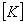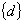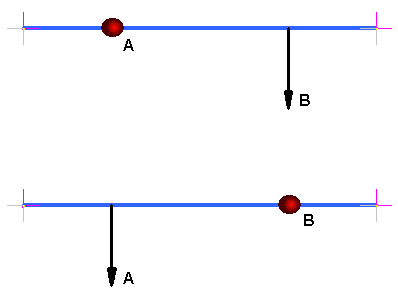#### Strand7 Software:  In Detail:  Solvers:  Load Influence

The Load influence solver is used to determine the influence that a point load or point moment has on a specified response variable. For example, if a beam axial force response variable is assigned, the Load Influence solver will establish the effect that a point force or point moment at any node in the model has on this axial force.

The Load Influence Solver performs the following steps:
• Calculates and assembles the element stiffness matrices and external nodal force vectors required to enforce each specified response variable. Constraints are also assembled in this process.

At the end of this assembly procedure, the following linear system of equilibrium equations is formed:whereGlobal stiffness matrixUnknown nodal influence vector(s)Global nodal load vector(s) resulting from each response variable. There is one virtual load case for each response variable.

• Solves the equations of equilibrium for the unknown nodal influence variables.

Theory
The load influence solver works on the principal of Maxwell's Reciprocal Theorem. Coupled with response variables for all elements, influence lines and influence surfaces can be extracted.

Maxwell's Reciprocal Theorem states:
For two identical forces at points B and A on a linear elastic structure as shown below:The displacement at A caused by a unit force at B, is the same as the displacement at B caused by a unit force at A. This is identical to stating that all stiffness matrices relating displacement to forces are symmetric.

In a more general form the Reciprocal Theorem can apply to all structural causes and effects. Thus in any linear structure the effect on any response variable at A, be it a moment, a rotation, a shear force, due to a unit force at B is the same as the response variable at B due to unit force at A.

Using this theory, the load influence solver determines the effect a unit load has on a particular response variable in the model, e.g. what displacement at point B will eventuate from a unit load at point A. All load and freedom case attributes are ignored for this solver except for response variables and restraints.

Any number of response variables can be solved for in each load influence analysis. Each response variable will produce a result case in the load influence results.

### Notes

1. The Displacement Scale can be used on an Influence solution to help the user visualise the results. However, the displaced model is not physically meaningful.

2. When looking at the results of a load influence analysis all the usual result reporting tools are available, e.g. peek tool, graphs, etc. However there are two common methods of result reporting, influence lines and influence surfaces.